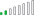cancel
Showing results for
Did you mean:Resolver II

## Performance issue using calculate and SUMX

Hi All,

I am having a table visual and I am seeing slow performance while adding the measure "test_acc" and it seems to load very slow.

test_acc =
var test_1 = calculate('Sales'[Volumes], left('Product'[Code],3)="ACC")
RETURN
calculate(SUMX('Sales', test_1 ),'Calendar'[cytd_flag]=1)

Please let me know how can i improve my formula if any great suggestions?
2 ACCEPTED SOLUTIONSSuper User

@alexa_0028 , try like

test_acc =
var test_1 = calculate(sum('Sales'[Volumes]), left('Product'[Code],3)="ACC")
RETURN
calculate( test_1,'Calendar'[cytd_flag]=1)

or

test_acc =
var test_1 = calculate(sum('Sales'[Volumes]), filter('Product', left('Product'[Code],3)="ACC"))
RETURN
calculate( test_1,filter('Calendar','Calendar'[cytd_flag]=1))Resolver II

Hi All,

With some retrying, I figured out the way to optimise. The formula I pasted above indeed worked, I was looking the performance along with old measures therefore it was showing high values.
Thank you so much @amitchandak  for pointing me to right direction.

test_acc =
var test_1 = calculate('Sales'[Volumes],KEEPFILTER( left('Product'[Code],3)="ACC") ,KEEPFILTER('Calendar'[cytd_flag]=1))
RETURN
test_1

4 REPLIES 4Resolver II

Hi All,

With some retrying, I figured out the way to optimise. The formula I pasted above indeed worked, I was looking the performance along with old measures therefore it was showing high values.
Thank you so much @amitchandak  for pointing me to right direction.

test_acc =
var test_1 = calculate('Sales'[Volumes],KEEPFILTER( left('Product'[Code],3)="ACC") ,KEEPFILTER('Calendar'[cytd_flag]=1))
RETURN
test_1Resolver II

I tried to rewrite my measure like below , it didn't improve performance much but gave right result:

test_acc =
var test_1 = calculate('Sales'[Volumes], left('Product'[Code],3)="ACC" ,'Calendar'[cytd_flag]=1)
RETURN
test_1Resolver II

Hi @amitchandak ,

But this solution doesn't give me the right results. I am looking for the results like highlighted in green but I am getting the value of the volume measure only in test_accSuper User

@alexa_0028 , try like

test_acc =
var test_1 = calculate(sum('Sales'[Volumes]), left('Product'[Code],3)="ACC")
RETURN
calculate( test_1,'Calendar'[cytd_flag]=1)

or

test_acc =
var test_1 = calculate(sum('Sales'[Volumes]), filter('Product', left('Product'[Code],3)="ACC"))
RETURN
calculate( test_1,filter('Calendar','Calendar'[cytd_flag]=1))